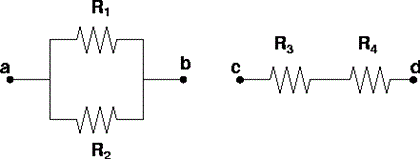# Problem: Consider the sections of two circuits illustrated above. Select True or False for all statements.1. After connecting a and b to a battery, (a) the voltage across R1 always equals the voltage across R2.(b) Rab is always less than or equal to R3.2. After connecting c and d to a battery, (a) the current through R3 always equals the current through R4.(b) Rab is always less than or equal to R1.

###### FREE Expert Solution

1.

The resistors in circuit section ab are in parallel.

The voltage across parallel resistors is the same.

83% (256 ratings)###### Problem DetailsConsider the sections of two circuits illustrated above. Select True or False for all statements.

1. After connecting a and b to a battery,

(a) the voltage across R1 always equals the voltage across R2.
(b) Rab is always less than or equal to R3.

2. After connecting c and d to a battery,

(a) the current through R3 always equals the current through R4.
(b) Rab is always less than or equal to R1.